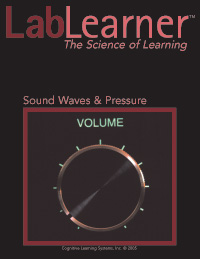# Sound Waves & PressureSound Waves & Pressure

Students investigate sound waves and the pressure waves that are responsible for the generation of sound. Investigate how vibrations in matter are the result of standing waves, how vibrations in one object can cause vibrations in another object and how these vibrations are longitudinal pressure waves. Students determine the speed of sound in air and investigate the inverse relationship between wavelength and frequency. Finally, students model the speed of sound in a liquid and a solid.

Investigation focus:

• Recognize that sounds are produced by vibrating matter occurring in solids, liquids or gases.
• Determine that the vibrations in objects are standing waves and that they consist of nodes and antinodes.
• Observe that vibrations can be transferred from one vibrating object to another.
• Understand that a sound is characterized by a wavelength and a frequency.
• Demonstrate that a specific sound is characterized by a specific frequency of vibrations.
• Determine the speed of sound in air.
• Realize that the speed of sound is the same in the same type of matter.
• Model the change in the speed of sound in different types of matter.
• Model that in different types of matter, the frequency remains constant but the wavelength changes.
• Use an equation relating the speed of sound to the frequency and the wavelength.
• Demonstrate the reciprocal relationship between the frequency and the wavelength of sound.